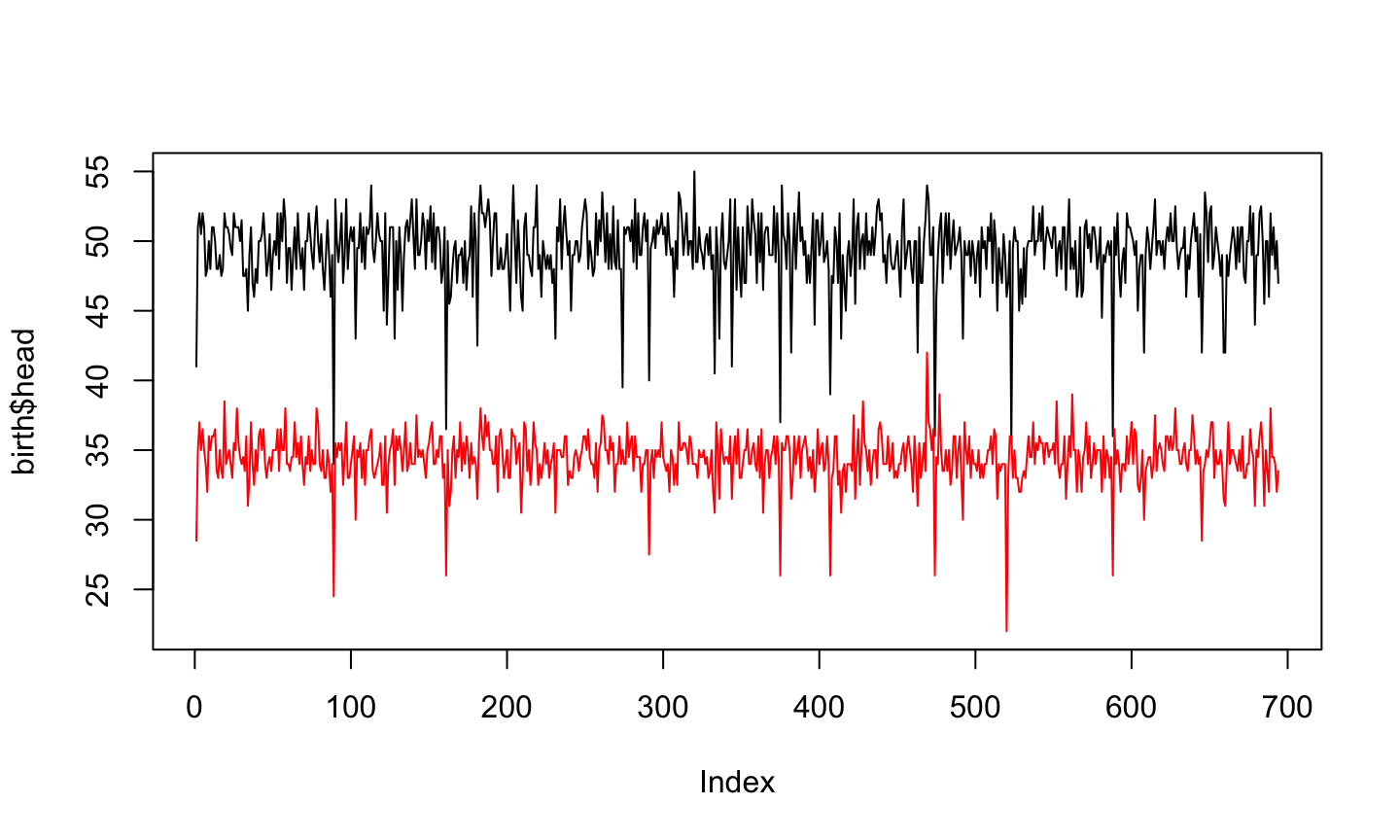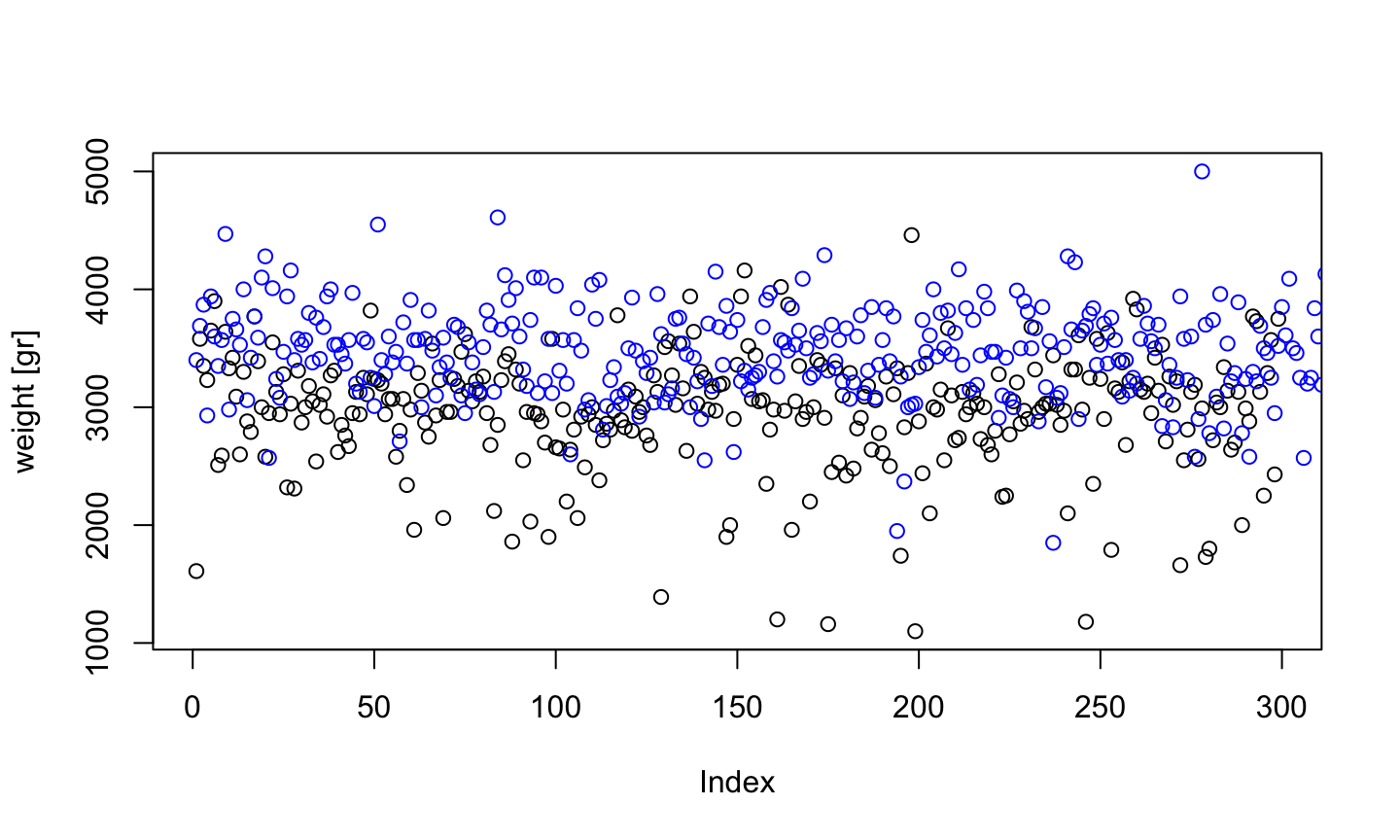## Telling Stories

Let’s recapitulate. We read data with

birth <- read.table("birth.txt", header=T)

which results in a data frame like this:

head(birth)
    id birth apgar5 sex weight head  age parity weeks
1 4347     1      8   F   1610 41.0 28.5      1    31
2 4346     1      9   F   3580 51.0 35.0      1    39
3 4300     1      9   F   3350 52.0 37.0      1    40
4 4345     1      9   F   3230 50.5 35.0      1    38
5 4349     1      8   F   3650 52.0 36.5      1    40
6 4315     2      8   F   3900 51.0 35.0      1    38

## In summary

summary(birth)
       id            birth           apgar5      sex         weight
Min.   : 4199   Min.   :1.000   Min.   :1.000   F:299   Min.   :1100
1st Qu.: 6112   1st Qu.:1.000   1st Qu.:8.000   M:395   1st Qu.:2972
Median : 7920   Median :1.000   Median :9.000           Median :3250
Mean   : 7877   Mean   :1.677   Mean   :8.281           Mean   :3244
3rd Qu.: 9606   3rd Qu.:2.000   3rd Qu.:9.000           3rd Qu.:3580
Max.   :11475   Max.   :3.000   Max.   :9.000           Max.   :5000
Min.   :34.0   Min.   :22.00   Min.   :1.000   Min.   :26.00
1st Qu.:48.0   1st Qu.:33.50   1st Qu.:1.000   1st Qu.:38.00
Median :50.0   Median :34.50   Median :2.000   Median :39.00
Mean   :49.3   Mean   :34.42   Mean   :2.611   Mean   :38.75
3rd Qu.:51.0   3rd Qu.:35.50   3rd Qu.:4.000   3rd Qu.:40.00
Max.   :55.0   Max.   :42.00   Max.   :9.000   Max.   :42.00  

What are these values?

## Extrema

The easiest to understand are minimum and maximum

min(birth$weight)  1100 max(birth$weight)
 5000

Which sometimes can be useful together

range(birth$weight)  1100 5000 ## Median If m is the median of a vector v, then • half of the values in v are smaller than m • half of the values in v are bigger than m median(birth$weight)
 3250

The median is the value $$m$$ minimizes the absolute error $$\sum_{i=1}^n \vert v_i-m\vert$$.

What if the number of elements is even?

## Quartiles

Quart means one fourth in latin.

If we split the set of values in four subsets of the same size

Which are the limits of these sets?

$$Q_0$$: Zero elements are smaller than this one
$$Q_1$$: One quarter of the elements are smaller
$$Q_2$$: Two quarters (half) of the elements are smaller
$$Q_3$$: Three quarters of the elements are smaller
$$Q_4$$: Four quarters (all) of the elements are smaller

It is easy to see that $$Q_0$$ is the minimum, $$Q_2$$ is the median, and $$Q_4$$ is the maximum

## Quartiles and Quantiles

Generalizing, we can ask, for each percentage p, which is the value on the vector v which is greather than p% of the rest of the values.

The function in R for that is called quantile()

By default it gives us the quartiles

quantile(birth$weight)  0% 25% 50% 75% 100% 1100.0 2972.5 3250.0 3580.0 5000.0  quantile(birth$weight, seq(0, 1, by=0.1))
  0%  10%  20%  30%  40%  50%  60%  70%  80%  90% 100%
1100 2630 2906 3020 3140 3250 3388 3530 3660 3850 5000 

## Arithmetic Mean

The mean value of the vector v is $\mathrm{mean}(v) = \frac{1}{n}\sum_{i=1}^n v_i$ where $$n$$ is the length of v. Sometimes it is written as $$\bar{v}$$

mean(birth$weight)  3243.905 This value is usually called average, but the correct name is mean This value minimizes the quadratic error $$\sum_{i=1}^n (v_i-\bar{v})^2$$ ## Mean of quadratic error if $$n$$ is the length of $$v$$ and $$\bar{v}$$ is its mean, then the mean of the quadratic error is $\frac{1}{n}\sum_{i=1}^n (v_i-\bar{v})^2$ This number is called variance of the sample This is a number in squared units, so it is hard to compare with the mean value ## Variance and Standard Deviation mean(birth$weight)
 3243.905
var(birth$weight)  273438 To understand easily the variability of the data, we take the square root of the variance The standar deviation of the sample is the square root of the variance $\sqrt{\frac{1}{n}\sum_{i=1}^n (v_i-\bar{v})^2}$ ## Variance and Standard Deviation In many cases, including in R, people uses a slightly different formula $\mathrm{sd}(v) = \sqrt{\frac{1}{n-1}\sum_{i=1}^n (v_i-\bar{v})^2}$ Explaining the reason is for a next course. (It is because of the bias of the expected value of the expected value) This value is called standard deviation of the population ## Variance and Standard Deviation The difference is small, especially when $$n$$ is big v <- birth$weight
sd(v)
 522.913
sqrt(sum((v-mean(v))^2)/length(v))
 522.5361
sqrt(sum((v-mean(v))^2)/(length(v)-1))
 522.913

## Graphics

Sometimes the best way to tell the story of the data is with a graphic

plot(birth$weight)each individual has a position in the x axis ## Another plot(birth$head)## Ploting Factors

The previous graphics used numeric data. What about factors?

plot(birth$head, type = "n", xlim=c(1,100))## Full annotation plot(birth$weight, main = "Weight at Birth", sub = "694 samples", ylab="weight [gr]")## Two plots in parallel

plot(birth$head) points(birth$age, pch=2)The first one defines the scale

## Two lines in parallel

plot(birth$head, type="l", ylim = c(22,55)) lines(birth$age, col="red")## Boys and Girls

plot(birth$weight[birth$sex=="F"], ylim=range(birth$weight), ylab = "weight [gr]") points(birth$weight[birth\$sex=="M"], col="blue")